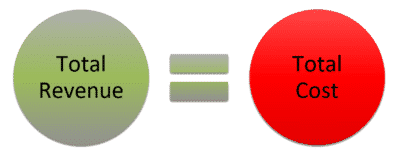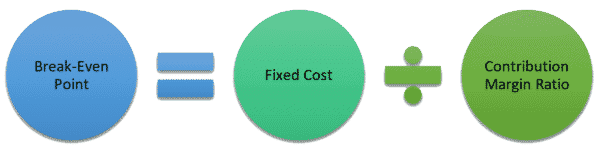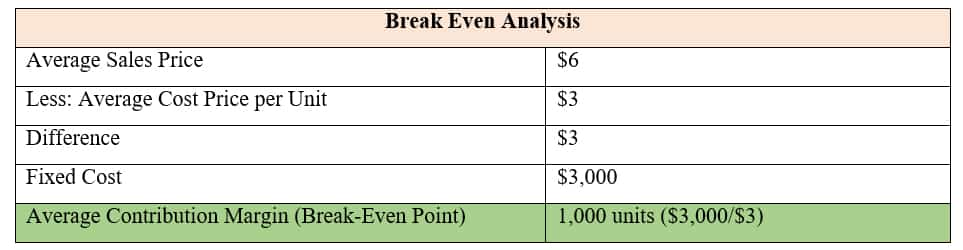## Find Out How to Calculate the Break-Even Point for a Restaurant

The break-even point is an important figure in running a profitable restaurant. It represents the number of sales your business needs to make over a period of time to remain profitable. It is the point at which your restaurant will not lose any money.  To give you a better understanding of this concept, this article will explain:

• What is the break-even point for a restaurant?
• How to calculate the break-even point for a restaurant?
• What is the difference between fixed and variable Costs?
• What is break-even analysis?

### What is the break-even point for a restaurant?

For most business owners, they tend to wonder when their business will break even. While this is a crucial metric in running a restaurant, some people tend to overlook it. However, it is important to conduct a break-even analysis to understand how profitable your business is. This will help you set the right prices for your products and aim at reaching optimal sales volume.The break-even point is the point at which the revenue of your restaurant equals the costs. It represents the amount of revenue needed to cover the restaurant’s fixed and total variable costs over a specific time period. Once this figure has been calculated, you get to know the number of sales you need to make a profit. It lets you know the number of people you need to serve at a determined average price point for your restaurant to make a profit.

### How do you calculate the break-even point for a restaurant?

The break-even point is usually calculated based on the industry. For businesses in the restaurant industry, the most effective way is using the average price and average cost of menu items. You start by separating the total fixed costs from the restaurant’s variable costs. The formula is as follows:

Break-even Point = Fixed Costs ÷ (Average Revenue per Menu Item – Average Cost per Menu Item)

When you calculate the break-even point for your restaurant, the units are the number of guests while the unit price is the dollar amount for the guest average. Since it is difficult to obtain the variable cost per guest, you can use estimates of your food and beverage margins in the following alternative formula.

Break-Even Point = Fixed Costs ÷ (Total Sales – Variable Costs/Sales)

The formula means that you divide your fixed costs with the contribution margin as follows:

Break-Even Point = Fixed Costs ÷ Contribution Margin RatioThe formula is effective in calculating your break-even point in a dollar amount. If you have categorized your costs into fixed and variable, you do not have to factor in the dollar average for each guest.

Breaking Down the Formula

The simplest way to understand the break-even point is that it is the number of menu items that your business needs to sell for your restaurant’s total costs to equal your revenue. Breaking down the formula, you calculate this point in the following five steps:

1. Calculate the total fixed costs. Add up all the costs that do not fluctuate on a monthly basis to get the total fixed costs.
2. Add up the costs of menu items, then divide the total with the number of items. The figure that you get is the average revenue per item.
3. Get the total cost of ingredients for every menu item. Then divide this figure with the total number of menu items to get the average variable cost.
4. Calculate the difference between the average revenue and the average cost to get the contribution margin.
5. Diving your total costs by the contribution margin to get your break-even point. The figure you get at this point represents the number of items in your menu that you need to sell monthly to break even.

### What is the Difference Between Fixed and Variable Costs?

Before you determine your break-even profit for your restaurant, you need to understand the difference between fixed and variable costs. Fixed costs are the expenses you must incur in your restaurant, regardless of your sales or production volume. They include licenses and permits, occupancy expenses, marketing costs, and communication tools like the internet. On the other hand, variable costs vary based on changes in production. They include food and beverage costs, disposables and garbage bags, cleaning supplies, prime costs, labor costs, and others. If the costs include fixed elements but are influenced by sales to some degree, then they are known as mixed costs.

### What is break-even analysis?

Break-even analysis allows you to make sense of the calculated figure.If your average sales price per unit is \$6 and your average cost per unit is \$3, then the difference between the two is \$3. If your fixed costs for the month are \$3,000, then your average contribution margin is \$3,000/\$3 = 1,000 units. This means that your restaurant will only make a profit if you make more than 1,000 units of sales per month. If the number of units sold in a month is less, then you suffer a loss.

Conclusion

The importance of calculating the break-even point is to determine the number of products your restaurant needs to sell to make a profit. As you conduct your break-even analysis you should aim at selling more products. You will also be able to determine whether the business is making profits under the current sale volumes.